Get inspired by the success stories of our students in IIT JAM MS, ISI  MStat, CMI MSc DS.  Learn More

# ISI MStat PSB 2014 Problem 1 | Vector Space & Linear TransformationThis is a very beautiful sample problem from ISI MStat PSB 2014 Problem 1 based on Vector space and Eigen values and Eigen vectors . Let's give it a try !!

## Problem- ISI MStat PSB 2014 Problem 1

Let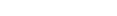where n is an odd positive integer. Let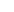be
the vector space of all functions from E to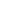, where the vector space
operations are given by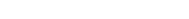, for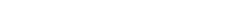for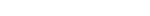(a) Find the dimension of(b) Let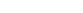be the map given by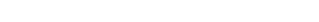Show that T is linear.
(c) Find the dimension of the null space of T.

### Prerequisites

Linear Transformation

Null Space

Dimension

## Solution :

While doing this problem we will use a standard notation for vectors of canonical basis i..e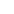. In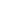they are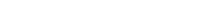and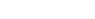.

(a) For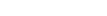and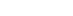, let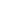be the function inwhich maps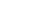and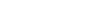where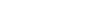and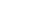. Then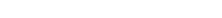is a basis of.

It looks somewhat like this ,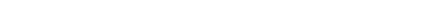,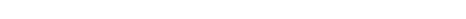Hence , dimension ofis 3n.

(b) To show T is linear we have to show that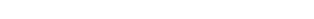for some scalar a,b .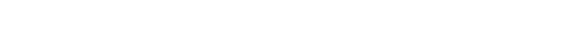.

Hence proved .

(c)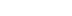gives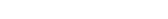so, the values of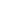for the last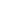points are opposite to first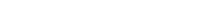so we can freely assign the values of f for firstto any of.Hence, the null space has dimension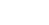## Food For Thought

let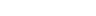be a non singular linear transformation.Prove that there exists a line passing through the origin that is being mapped to itself.

Prerequisites : eigen values & vectors and Polynomials

## Subscribe to Cheenta at Youtube

This is a very beautiful sample problem from ISI MStat PSB 2014 Problem 1 based on Vector space and Eigen values and Eigen vectors . Let's give it a try !!

## Problem- ISI MStat PSB 2014 Problem 1

Letwhere n is an odd positive integer. Letbe
the vector space of all functions from E to, where the vector space
operations are given by, forfor(a) Find the dimension of(b) Letbe the map given byShow that T is linear.
(c) Find the dimension of the null space of T.

### Prerequisites

Linear Transformation

Null Space

Dimension

## Solution :

While doing this problem we will use a standard notation for vectors of canonical basis i..e. Inthey areand.

(a) Forand, letbe the function inwhich mapsandwhereand. Thenis a basis of.

It looks somewhat like this ,,Hence , dimension ofis 3n.

(b) To show T is linear we have to show thatfor some scalar a,b ..

Hence proved .

(c)givesso, the values offor the lastpoints are opposite to firstso we can freely assign the values of f for firstto any of.Hence, the null space has dimension## Food For Thought

letbe a non singular linear transformation.Prove that there exists a line passing through the origin that is being mapped to itself.

Prerequisites : eigen values & vectors and Polynomials

## Subscribe to Cheenta at Youtube

This site uses Akismet to reduce spam. Learn how your comment data is processed.

### Knowledge Partner